Share

# Construct Trianglepqr If Pq = 5 Cm, M∠Pqr = 105° and M∠Qrp = 40°. (Hint: Recall Angle Sum Property of a Triangle). - CBSE Class 7 - Mathematics

ConceptConstructing a Triangle When the Measures of Two of Its Angles and the Length of the Side Included Between Them is Given. (ASA Criterion)

#### Question

Construct ΔPQR if PQ = 5 cm, m∠PQR = 105° and m∠QRP = 40°. (Hint: Recall angle sum property of a triangle).

#### Solution

A rough sketch of the required ΔPQR is as follows.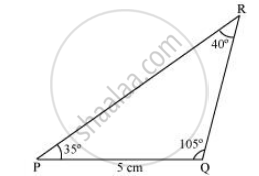In order to construct ΔPQR, the measure of ∠RPQ has to be calculated.

According to the angle sum property of triangles,

∠PQR + ∠PRQ + ∠RPQ = 180º

105º + 40º + ∠RPQ = 180º

145º + ∠RPQ = 180º

∠RPQ = 180° − 145° = 35°

The steps of construction are as follows.

1) Draw a line segment PQ of length 5 cm.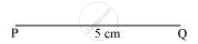2) At P, draw a ray PX making an angle of 35º with PQ.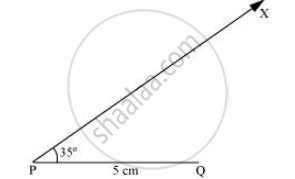3) At point Q, draw a ray QY making an angle of 105º with PQ.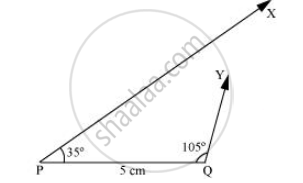4) Point R has to lie on both the rays, PX and QY. Therefore, R is the point of intersection of these two rays.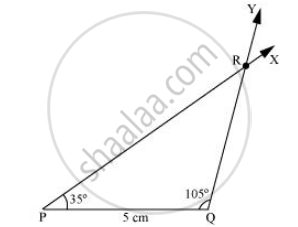This is the required triangle PQR.

Is there an error in this question or solution?

#### APPEARS IN

NCERT Solution for Mathematics for Class 7 (2018 to Current)
Chapter 10: Practical Geometry
Ex. 10.40 | Q: 2 | Page no. 202
Solution Construct Trianglepqr If Pq = 5 Cm, M∠Pqr = 105° and M∠Qrp = 40°. (Hint: Recall Angle Sum Property of a Triangle). Concept: Constructing a Triangle When the Measures of Two of Its Angles and the Length of the Side Included Between Them is Given. (ASA Criterion).
S